# Extension theorems

Theorems on the continuation (extension) of functions from one set to a larger set in such a way that the extended function satisfies certain definite properties. Problems on the analytic continuation of functions are, first of all, related to extension theorems.

An example of a theorem on the existence of a continuous extension of a continuous function is the Brouwer–Urysohn theorem: Ifis a closed subset of a normal spaceand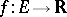is a continuous real-valued bounded function, then there exists a continuous bounded functionsuch that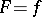on. The Hahn–Banach theorem on the extension of linear functionals in vector spaces is an extension theorem.

In a Euclidean space extension theorems are mainly related to the following two problems: 1) the extension of functions with domain properly belonging to a space onto the whole space; and 2) the extension of functions from the boundary to the entire domain. In both cases it is required that the extended function has definite smoothness properties, i.e. belongs to an appropriate class of functions, depending on the properties of the function to be extended.

The problem of extending functions from a domain with a sufficiently smooth boundary to the whole space while preserving continuity of the partial derivatives was solved by M.R. Hestenes  and H. Whitney . If functions,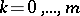, are given on the-dimensional boundary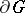of a domainin the-dimensional space, then the problem of constructing a functionfor which(*)

whereis the normal to, has been considered by E.E. Levi , G. Giraud ,

and M. Gevrey  in case the smoothness of theand ofis described in terms of continuity and membership of a Hölder space (in the presence of, possibly, certain singularities). The order of growth of the partial derivatives of ordersas the argument tends to the boundaryofhas also been studied.

Both problems have been systematically studied by S.M. Nikol'skii and his students (cf. , ) in the cases of extension of functions in various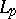metrics, in various variations and in various function spaces. Best characteristics of differentiability properties of functions that can be obtained from extending functions with given differentiability-difference properties have been found in terms of series of function spaces (cf. Imbedding theorems). Concerning the problem (*) one has found extensions that are optimal with respect to the order of growth of the derivatives of orders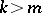when approaching the boundary of the manifold (cf. , ).

Often one substantiates methods for extending functions and systems of functions (*) from the boundary to the whole domain by integral representations. Usually, convenient methods for the extension of functions are linear. There are also other methods, e.g. based on expanding functions in series with subsequent extension of each term of the series. This method is, as a rule, non-linear. There are cases in which a linear method definitely does not exist, .